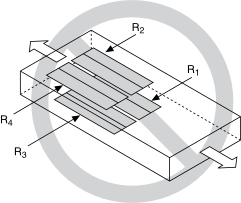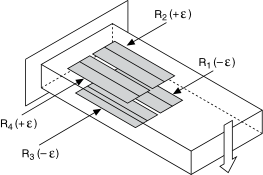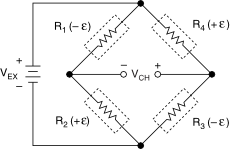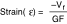# Full-Bridge Type I

## NI-DAQmx 18.6 Help

Edition Date: January 2019
Part Number: 370466AH-01
View Product InfoNI-DAQmx 18.6 HelpNI-DAQmx 19.0 HelpNI-DAQmx 19.1 HelpNI-DAQmx 19.5 Help

The full-bridge type I configuration only measures the bending strain.The following figure shows how to position strain gage resistors in a bending configuration for the full-bridge type I.Full-bridge type I strain gage configurations have the following characteristics:
• Four active strain gage elements, two mounted in the direction of bending strain on the top side of the strain specimen and the other two mounted in the direction of bending strain on the bottom side.
• High sensitivity to bending strain.
• Rejection of axial strain.
• Compensation for temperature.
• Sensitivity at 1000 µε is ~ 2.0 mVout / VEX input.
Related concepts

## Full-Bridge Type I Circuit DiagramThe following symbols apply to the circuit diagram:
• R1 is the active strain gage element measuring compressive strain (–ε).
• R2 is the active strain gage element measuring tensile strain (+ε).
• R3 is the active strain gage element measuring compressive strain (–ε).
• R4 is the active strain gage element measuring tensile strain (+ε).
• VEX is the excitation voltage.
• RL is the lead resistance.
• VCH is the measured voltage.

The following equation converts voltage ratios to strain units for full-bridge type I configurations.where Vr is the voltage ratio that virtual channels use in the voltage-to-strain conversion equation, and GF is the gage factor.